pH

pH is a very convenient scale to measure large variations of [H3O+] concentrations.

pH is defined by the expression belowThe above expression can be rearranged to give: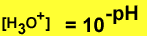Example1. Calculate the pH of a 0.1M HCl solution.
Step 1 find the concentration of hydronium ions. Since HCl is a strong acid the reaction represented by the equation below has a very high Ka value therefore the reaction proceeds almost to completion.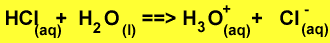The concentration of HCl should be equal to the concentration of hydronium ions.

Step 2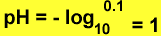Example 2. Calculate the concentration of hydronium ions in a sample of a lemon soda drink of pH 3.0.
Step 1 Apply the formula below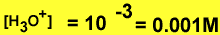Complete the table below
click in the coloured cells for more information. Double click to hide the information.

 Concentration of strong acid pH of solution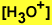2 0.001 0.002 9 0.2 0.1 0.01